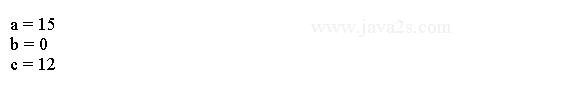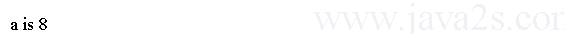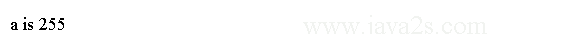# Java Tutorial - Java Bitwise Operators

Bitwise Operators act upon the individual bits of their operands. Java bitwise operators can be applied to the integer types: `long, int, short, char, byte`.

## Bitwise operators List

The following table lists all Java bitwise operators.

Operator Result
~ Bitwise unary NOT
& Bitwise AND
| Bitwise OR
^ Bitwise exclusive OR
>> Shift right
>>> Shift right zero fill
<< Shift left
&= Bitwise AND assignment
|= Bitwise OR assignment
^= Bitwise exclusive OR assignment
>>= Shift right assignment
>>>= Shift right zero fill assignment
<<= Shift left assignment

Bitwise operator assignments combines the assignment with the bitwise operation. The following two statements are equivalent:

```a = a >> 4;
a >>= 4;
```

The following two statements are equivalent:

```a = a | b;
a |= b;
```

The following program demonstrates the bitwise operator assignments:

```
public class Main {
public static void main(String args[]) {
int a = 1;/*from   ww w  .j a va  2 s.co  m*/
int b = 2;
int c = 3;
a |= 2;
b >>= 2;
c <<= 2;
a ^= c;
System.out.println("a = " + a);
System.out.println("b = " + b);
System.out.println("c = " + c);

}
}
```

The output of this program is shown here:## Java Left Shift Operator

The left shift operator, <<, shifts all of the bits in a value to the left a specified number of times.

It has this general form:

```value << num
```

The following code shifts byte type variable.

```
public class Main {
public static void main(String args[]) {
byte a = 64, b;
int i;/*from   ww  w.j a v a 2 s .c  o  m*/
i = a << 2;
b = (byte) (a << 2);
System.out.println("Original value of a: " + a);
System.out.println("i and b: " + i + " " + b);
}
}
```

The output generated by this program is shown here:## Example

Each left shift has the effect of doubling the original value. The following program illustrates this point:

```
public class Main {
public static void main(String args[]) {
int num = 0xFFFFFFF;
// ww  w  .j  a va 2 s.  com
for (int i = 0; i < 4; i++) {
num = num << 1;
System.out.println(num);

}
}
}
```

The program generates the following output:## Java Right Shift Operator

The right shift operator, `>>`, shifts all of the bits in a value to the right a specified number of times.

Its general form is shown here:

```value >> num
```

`num` specifies the number of positions to right-shift.

The following code fragment shifts the value `32` to the right by two positions:

```
public class Main {
public static void main(String[] argv) {

int a = 32;
a = a >> 2;
System.out.println("a is " + a);

}
}
```

The output:## Java Unsigned Right Shift

Java's unsigned, shift-right operator, `>>>`, always shifts zeros into the high-order bit.

Its general form is shown here:

```value >>> num
```

`num` specifies the number of positions to right-shift.

The following code shows how to use unsigned right shift.

```public class Main {
public static void main(String[] argv) {
int a = -1;
a = a >>> 24;

System.out.println("a is " + a);
}
}
```

The output: